Subsections

# Spectral Representation

## Definitions

We applied Fourier transform for space-dependent problems--and we were successful. Let us do the same for time-dependent problems: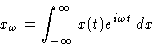(1)
Inverse transform: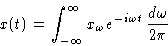## Correlation Function

The product of x's is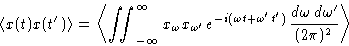Or, since only x's are random,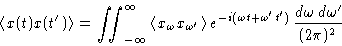Symmetry with respect to time: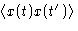depends only on t-t'. This means that in the integral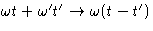. This means that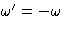.
Conclusion: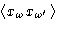should be proportional to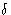-function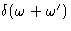Definition:
Let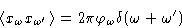(2)
Then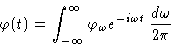We see that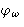is indeed the Fourier transform of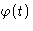. This is exactly the function measured in inelastic scattering, relaxation experiments (NMR, ESR, dielectric spectroscopy, shear rheometry,...). Inverse: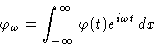If t=0, we obtain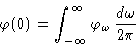But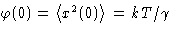. We obtained: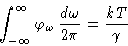## Relaxation in Fourier Space

We obtained for correlation function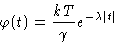Take Fourier transform: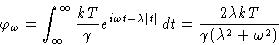(3)
This is a Lorentzian!

Relaxation with one characteristic time is Lorentzian.

Many characteristic times--more complex formulae.

## Example: Velocity Autocorrelation Function

Consider a large particle surrounded by small particles (a colloidal particle among molecules). Equation for average velocity: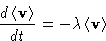with friction coefficient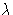. For a spherical particle with radius r and mass m in liquid with viscosity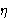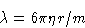(4)
The average square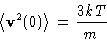We immediately obtain autocorrelation function:
• In the real time: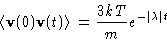• After Fourier transform: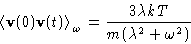(5)
Equations (3) and (5) are special cases of fluctuation-dissipation theorem.

In particular, if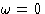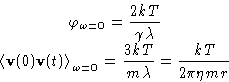## Example: Diffusion

Consider particles with concentration c. Diffusion equation with diffusion coefficient D:Fourier transform in space: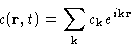We obtain: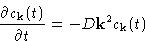Let us now consider equilibrium. In equilibrium the fluid is homogeneous. For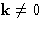: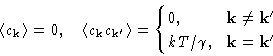with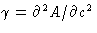.

We immediately obtain: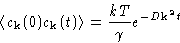Or, in Fourier space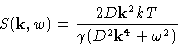This is dynamic structure factor.Next: Random Force and Its Up: Time Correlation Functions. Random Previous: Time Correlation Functions. Random

© 1997 Boris Veytsman and Michael Kotelyanskii
Tue Oct 28 22:16:24 EST 1997